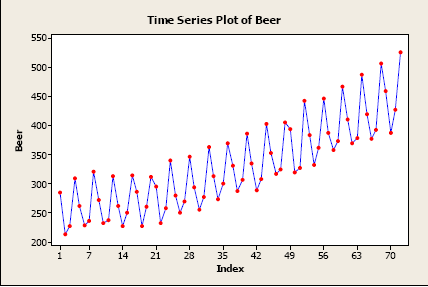## Tuesday, 16 August 2016

### Time Series and Fitting Regression on Time Series Data

Time Series and Fitting Regression on Time Series Data

Time series is one of the easiest topic in Data Science if understood conceptually. It can be related to standard regression. One difference from standard linear regression is that the data are not necessarily independent and not necessarily identically distributed.  One defining characteristic of time series is that this is a list of observations where the ordering matters.  Ordering is very important because there is dependency and changing the order could change the meaning of the data.
A univariate time series is a sequence of measurements of the same variable collected over time.  Most often, the measurements are made at regular time intervals. Any time series is assumed to be made of 4 components.

1. Secular Trend (or General Trend)
2. Seasonal Movements
3. Cyclical Movements
4. Irregular Fluctuations
General trend is long term effect of some external factor. Trend may show a growth or decline over a very long period of time. Seasonality is short term movement due to seasonal factors like demand of ice-creams in summer. Cyclicity or Business Cycle is simply a repetition of long term oscillation. Randomness or Irregularity is sudden changes which are unlikely to predict.Below Image shows a time series of quarterly production of beer in Australia for 18 years.Lets look at the component of above series. ( Image is taken from “Applied Time Series Analysis” course by Eberly College of Science). There is an upward trend. It is pretty obvious from the image. Another way of looking at the trend is to draw mean production line and then check if new data points are trying to come near the mean? If yes, there is no trend. There is seasonality as we can see regular repeating patterns in every year.

Fitting Regression model for above series-

Suppose that the observed series is x(t), for t=1,2,,n
.
• For a linear trend, use t (the time index) as a predictor variable in a regression.
• For a quadratic trend, we might consider using both t and t2.
• For quarterly data, with possible seasonal (quarterly) effects, we can define indicator variables such as Sj = 1 if observation is in quarter j of a year and 0 otherwise.  There are 4 such indicators.
Equation for fitting linear trend and seasonality would be x(t)= A*t +B* S1 +C*S2+D*S3+E*S4.
A -E are coefficients to be multiplied to independent variables; t,S1,S2,S3,S4. RMSE or R Square can be used for model validation. I would also like to mention that R square is a comparison parameter to know 'how better regression is fitting data points compare to simple average”. More variables like quadratic trend; t^2 etc can be added and then R square can be used to identify best model.

another beautiful article on anomaly detection is present at-

Anomaly Detection and it's types